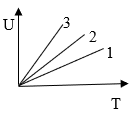Change in internal energy
Question

# The internal energy of  gases He, O2 and NH3 are plotted against the absolute temperature.  The respective graphs 1, 2  and 3 are ofEasy
Solution

## The internal energy  $\mathrm{U}=\frac{\mathrm{f}}{2}\mathrm{RT} \mathrm{and} {\mathrm{f}}_{{\mathrm{NH}}_{3}}>{\mathrm{f}}_{{\mathrm{O}}_{2}}>{\mathrm{f}}_{\mathrm{He}}$ where f is degrees of freedom

Get Instant Solutions